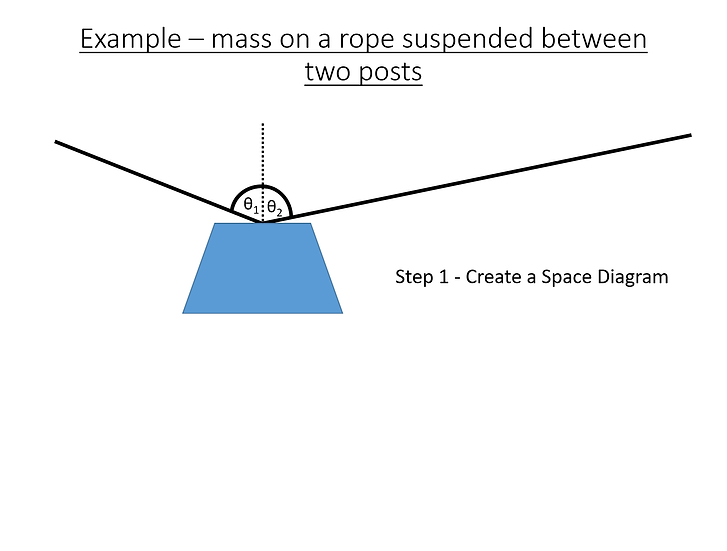# BTec Engineering - Vectors (PowerPoint and Worksheet)

£2.50Price
`This is an 12 slide PowerPoint that covers BTec National Engineering (A-Level equivalent) plus a worksheet and the answers to the worksheet This covers the mathematical and physics of vectors, resolving vectors and creating free body diagrams, space diagrams and vector diagrams This PowerPoint forms Lesson 1 in this series (Static Engineering Systems)`